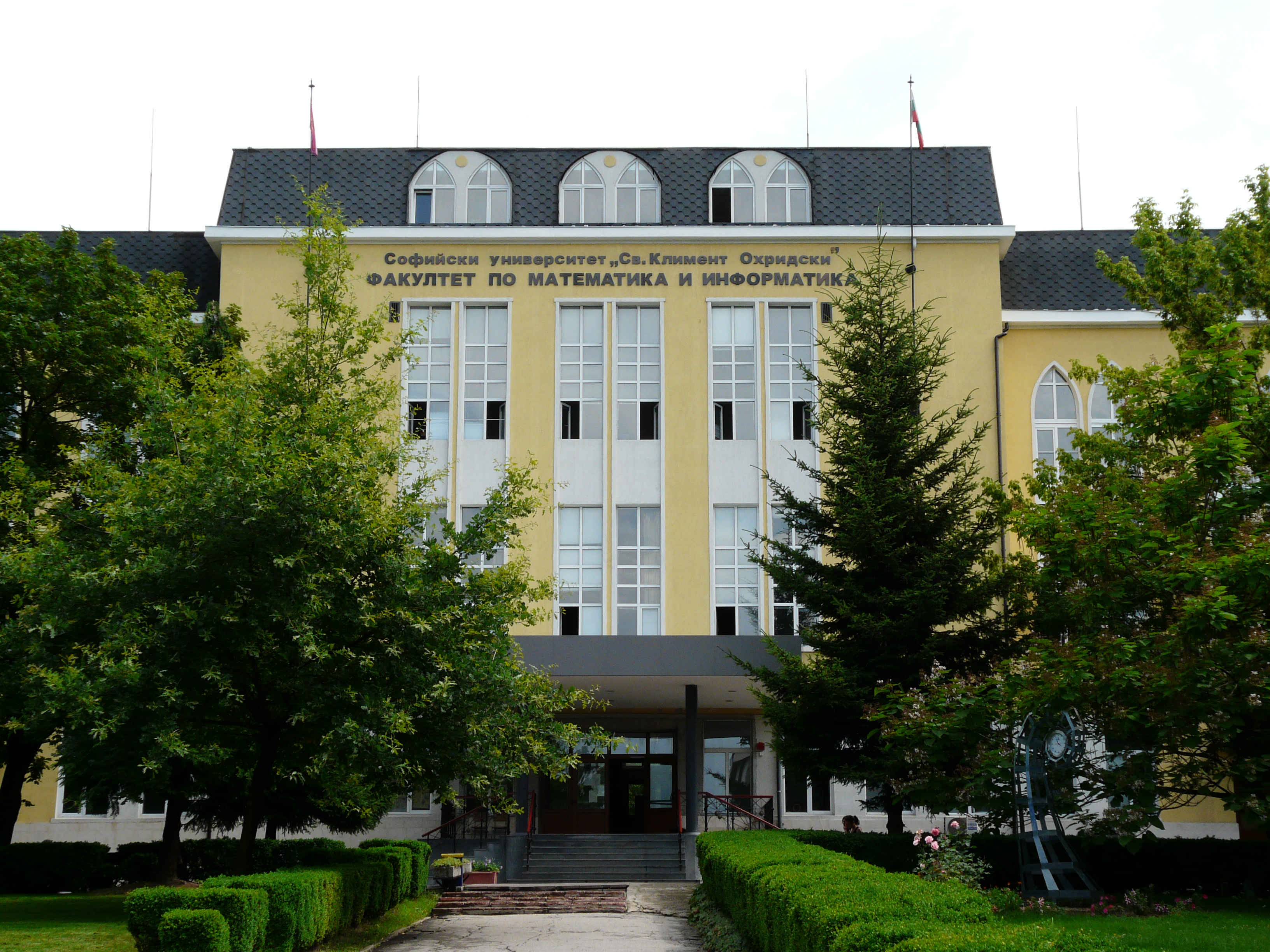# A successful direction of studies of the students at the MSc program “Computational Mathematics and Mathematical Modelling” at the Faculty of Mathematics and Informatics, Sofia UniversityThe MSc Program “Computational Mathematics and Mathematical Modelling” at the Faculty of Mathematics and Informatics at Sofia University is certified by ECMI as a Model Msc Program in Technomathematics. It gives a broad choice of courses in Mathematical Modelling, Numerical methods and fundamental mathematical disciplines, needed for working in the field of Applied and Industrial Mathematics.

One of the fields of application that are included in the MSc program concerns application of Mathematics to Biology and Biomedicine. There are several courses for students interested in Biomathematics and mathematical modelling in Biology. Also, regular talks are given at the Seminar in Mathematical Modelling by specialists in the field.

This has resulted in the recent years in increased interest of students to those disciplines and they get involved in research in the field. Some recent related MSc Theses, developed by our students include:

• Zdravka Nedyalkova, Multiphysics simulations of the process of neuromuscular activation

In this Master’s thesis, the problem of mathematical modelling and computer simulations of neuromuscular activation is considered. The biological and biochemical processes that result in a muscle contraction are described. For each of them, a mathematical model, established in the literature, is derived. In particular, the following are considered:

• the Hodgkin–Huxley model of neural transmission;
• a reaction-diffusion model for the process of neurotransmitter release in the synaptic gap between a nerve and a muscle cell;
• an ODE system, based on chemical reaction schemes, proposed by Williams, for the process of calcium dynamics inside the muscle cell;
• Hill’s model for the generation of muscle force, triggered by calcium dynamics.

The models are studied computationally and/or analytically. Further, a framework for coupling the models, mentioned above, is proposed so that new integrated multiphysics simulations of the whole process are obtained.

• Galina Lyutskanova, Mathematical modeling of anisotropic surfaces with elastic properties. Applications in red blood cell modelling

The mathematical modelling of elastic membranes is a very active field of research. It has wide spectrum of applications, e.g. theoretical description of biomembranes, synthetic capsules, etc. In this work,  a new mathematical model of the shape of an axisymmetric anisotropic membrane in mechanical equilibrium, based on the general two-dimensional elastic continuum model, is obtained. It can be formulated as a system of six first-order ordinary differential equations. A particular case of the model with zero bending moments is studies and applied to red-blood-cell-like materials. The model comprises of four nonlinear ordinary differential equations, which are solved numerically. To this end, an adaptive numerical method is proposed.

Finally, the problem of computing the equilibrium shape of an incompressible membrane is formulated as a minimization problem and solved using the Deepest descent method. The mathematical model is validated by showing that the obtained solutions are in accordance with known theoretical and experimental results.

• Gergana Velikova, Mathematical models, describing biological systems under the influence of inhibitory conditions

The MSc thesis is an extensive study of mathematical models of cell growth under inhibitory conditions in the medium. It describes and discusses the applicability of many existing and widely used models of cell growth (usually used in optimal conditions). Then, a new model, based on biological considerations and reaction schemes is derived. All models are validated on the basis of experimental data. Parametric identification is carried using classical numerical methods. Comparison and biological interpretation of the obtained parameters are made.

An interesting fact is that the diploma student has first graduated as a BSc in Biotechnology. This allowed her to carry out the biological experiments herself. Thus, the MSc thesis is highly interdisciplinary in its essence.

For other fields of interest of our students, we shall use another opportunity to tell.